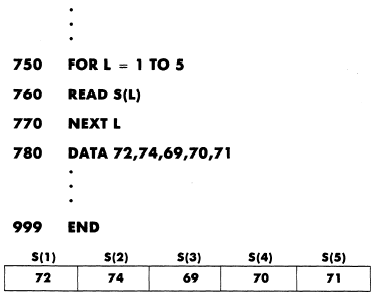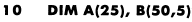Working with arrays
Custom SearchWORKING WITH ARRAYS

In BASIC, we have the capability to store and reference data elements in lists or tables. These are called arrays. An entire array is assigned one name (e.g., D), and yet, we can refer to any element in the array by using a subscripted variable. If D is such an array, then D(l), read "D sub one, " is the first element in array D. The value in parentheses is called a subscript. It indicates the relative position of a given element in an array. For example, LET D(3) = D(1) + D(2) is a BASIC statement which adds the first two numbers of array D and puts the sum in the third element of array D.

Before elements in arrays can be used, a method is needed to define the array. The DIM (DIMENSION) statement is used for this. It names the array and reserves memory space. For example, DIM D(15) would reserve space for 15 data elements with the name, D.

When subscripted variables are used, their corresponding values must be read and stored in memory during program execution. Examine the follow-ing example (one-dimensional array) and see how the data is stored in the computer’s memory when the program statements are executed.

Example:In this example, the loop will be executed five times. The variable used to control the loop is also used as the subscript for S in the READ statement. On the first pass through the loop, the subscript L is 1, the value of L during the first execution of the loop. On the second pass, the subscript will be 2, and so on, until the value of L is equal to 5.

Subscripted variables can also be used to identify elements in tables (two-dimensional arrays) but it takes two subscripts, one to specify the row and a second one to specify the column. For example, S(2,5) would specify the location of the value in the second row, fifth column. Figure 5-1 is an example of a two-dimensional array.

DIM (DIMENSION) Statement

As stated earlier a method is needed to specify the size of an array. The BASIC programming language automatically assigns 11 elements to every one-dimensional array and 121 elements (11 rows and 11 columns) to every two-dimensional array appearing in a program.

Larger arrays may be used. However, the size of each must be defined; that is, you must specify the maximum number of elements in each. The follow-ing example shows how a DIM statement is constructed.

Example:This DIM statement would reserve memory space for an array named A with 25 elements, and an array named B with 50 rows and 5 columns. On some computers the first element in a one-dimensional array is referenced with subscript 0, and in a two-dimensional array by subscripts, 0, 0. If that is the case on your computer, you would set the upper limits at one less than the number of elements you need.

When the BASIC interpreter encounters a DIM statement such as the one above, it reserves an area in memory for arrays A and B made up of 25 and 250 elements respectively.

If an array requires less storage space than is automatically reserved by the BASIC interpreter it need not be defined by a DIM statement. However, by using a DIM statement only the space actually needed will be reserved.

Arrays

Some important things to remember about arrays:

DIMENSION statements are used to define arrays.

The elements in an array can be either numeric quantities or strings.

However, all of the elements in a given array must be the same type (all numeric or all string).

An array that contains numeric elements must be named with a single letter.

A string array is referred to with a letter followed by a dollar sign.

Elements in a one-dimensional array are referenced by the array name followed by a subscript in parentheses, A(l).

Elements in a two-dimensional array, matrix, are referenced by the name followed by two subscripts in parentheses; the first References the row, the second the column, A(2,3).

Subscripts may be a numeric-constant or a numeric-variable.

Each array name in a program must be unique. However, an array and an ordinary variable can have the same name. Duplicating array names and variable names could be logically confusing; therefore, it is not a recommended practice.

Many computers have a special set of instructions called MAT instructions for working with matrices. By using a single MAT instruction, matrices may be defined, added, subtracted, multiplied, read, and manipulated in a variety of ways. Any of these operations may be done with FOR-NEXT loops; however, the MAT statement, if available, makes it easier. See Appendix II for examples and refer to the user’s manual for your specific computer.Integrated Publishing, Inc. - A (SDVOSB) Service Disabled Veteran Owned Small Business